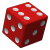Games
Problems
Go Pro!

# Probability

Reference > MathematicsA brief explanation of probability with examples of different probability valuesA simple mathematical formula for determining a probability for a single eventMutually exclusive outcomes are outcomes that can't both happen simultaneouslyAdding probabilities to obtain the probability of two mutually exclusive eventsHow to calculate the probability of outcomes which are not mutually exclusiveTwo outcomes are complementary if they are the only possible outcomesIndependent and dependent events - what they are, how to recognize the differenceFinding the probability of compound events (two events happening together or in succession)Like us on Facebook to get updates about new resources